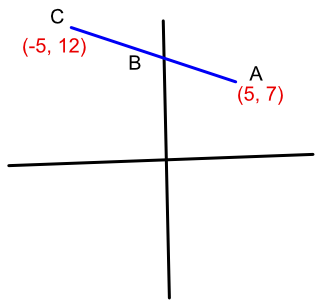Parallel Vectors

## Parallel Vectors

A vector ((a),(b)) may be a #position vector# which describes a vector from the origin O to a point (a, b).

Parallel vectors are vectors that have the same direction but may have different magnitude. In the diagram, below, vectors a and b are parallel, and a = 2b. The multiplier is known as a scalar.In the diagram below, points A, B and C are on the same line (they are said to be collinear). Vector vec(AB) = kvec(AC), where k is a scalar.## Example 1

Show that points A at (3, 5), B at (7, 11) and C at (13, 20) are collinear.

The explanation as well as the calculation should be provided.

Answer: vec(AB) = ((4),(6)) and vec(AC) = ((10),( 15))

Let bb(a) = vec(AB) and bb(b) = vec(AC)

bb(a) = 2.5bb(b)

a is a multiple of b and both share at least one point; they are collinear.

## Example 2

Three points are on a straight line AC. Point A is at position (5, 7). Point C is at (-5, 12). Given vec(AC) = bb(a) and vec(AB) = bb(b), and bb(b) = frac(3)(5)bb(a), what is the position of point B?

vec(AC) = ((-10),(5))

vec(AB) = frac(3)(5) xx ((-10),(5)) = ((-6),(3))

Question asks for the position of point B;

Origin to point A + vector ((-6),(3)) is (5 - 6, 7 + 3) = (-1, 10)

Use a quick sketch if that helps: